## Introduction

The fast uptake of renewable sources in power generation in the recent years has aggravated the problem of flexibility of conventional power generation systems. The intermittent nature of the renewable sources (solar and wind in particular) imposes new operational challenges for power plants which will be required to ramp more often with frequent shut-downs and start-ups. During the shut-down regimes, the turbine casing components are exposed to the buoyant flows with pure natural and mixed convection, which then lead to non-uniform cooling due to temperature non-uniformity on the components. Consequently, the non-uniform cooling rates may cause severe fatigue on the casing.

Considering these challenges, the first step towards addressing the issues would be ensuring that the flow physics during the shut-down regimes are thoroughly investigated. The experimental investigations, however, are often not feasible since the direct measurements in the flow field in the turbine cavities are not trivial. Therefore, majority of the observations heavily rely on computational methods. Although a large body of literature has sought to provide experimental data for pure natural (for example Beckmann (1931), Liu et al. (1961), Grigull and Hauf (1966), Kuehn and Goldstein (1976), and Fahy et al. (2018)) and forced convection (Brighton (1963), Farias et al. (2009) and Hosseini et al. (2009) among others), mixed type of convection in the turbine casing cavities has not been previously assessed to a broad extent. The experimental investigations concerning the mixed type type of flows have been mostly on fully developed duct and pipe flows (for example Kotake and Hattori (1985), Ciampi et al. (1987) and Mohammed et al. (2010)). In the recently conducted research of Murat et al. (2021), a unique experimental facility has been designed and commissioned for mixed type of flows in turbine casing cavities during shut-down regimes, and also incapabilities of Reynolds-averaged Navier-Stokes (RANS) in predicting these type of flows have been highlighted by demonstrating the inadequacy of isotropic turbulence assumption.

The unavailability of the extensive experimental data, in return, ultimately reduces the confidence in the numerical results, which hinders the design optimization and the advance turbulence modelling development for the mixed type of flows.

## Experimental methodology

### Background

Mixed type of convection occurs in a wide range of turbomachinery applications, and are therefore of great importance. As mentioned earlier, especially high pressure (HP) steam turbine casing is exposed to both natural and mixed convection during shut-down regimes with different operating conditions. The cavity formed by the inner and outer turbine casings could be considered as a horizontal concentric annulus as seen in Figure 1 where various flow regimes taking place in the casing cavity are also presented.

### Variation of flow regimes in casing cavities.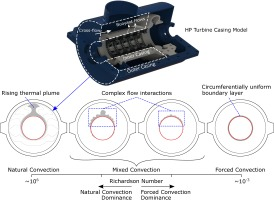In the case of pure natural convection, thermal plume occurs at the top of the heated inner cylinder, which rises and impinges on the outer casing. This leads to a non-uniform cooling. After impingement, the fluid cools down and moves down the lower portion of the annulus where the flow tends to be stable. When the pure forced convection takes places, a circumferentially uniform boundary layer forms around the inner cylinder as shown in Figure 1. The flow behaviour in the upper portion of the annulus significantly changes when both natural and forced convection take place simultaneously. The thermal plume region is distorted by the cross-flow, and multiple longitudinal structures develop due to the complex flow interactions.

### Experimental facility

An experimental facility illustrated in Figure 2 has been designed for the detailed investigations of mixed (natural and forced) convective type of flows in a simplified turbine casing cavity represented by a horizontal concentric annulus. The inner casing temperature is kept constant by a PID controller at a set value, while the incoming air speed is varied by an industrial blower at the outlet for different mixed convection rates. Low conductivity calcium silicate inserts are used at both end of the inner cylinder, which is made of copper, to minimise the heat loss. Self-adhesive ceramic heat shields have been placed around the outer casing cylinder to reduce the heat dissipation. The facility consists of various measurement ports distributed axially and circumferentially. Measurement locations on the test facility are depicted in the facility schematic in Figure 3 and some of the essential dimensions are tabulated in Table 1. The detailed design information regarding the experimental facility can be found in the study of Murat et al. (2021).

### Cross-section of the test facility CAD model.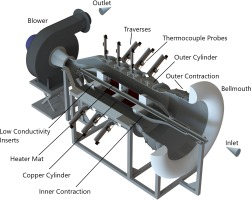### Experimental facility schematic and measurement locations.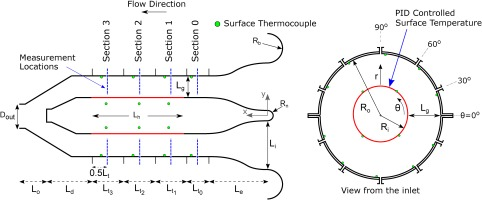### Rig dimensions.

ParameterDimension (mm)ParameterDimension (mm)ParameterDimension (mm)ParameterDimension (mm)
Ri180Rn70Le720Ld425
Ro400Li440Lt0250Lo390
Rb150Lg220Lt1,2,3300Dout200

For pure natural and forced convective flows, Grashof and Reynolds numbers are important non-dimensional parameters to determine the flow regime. Richardson number is used when both natural and forced convection take place simultaneously. The non-dimensional numbers used in the present study are given in Equation 1.

##### (1)
GrLg=gβ(TT)Lg3ν2,ReDh=uDhν,Ri=GrRe2

It is important that a test facility is able operate at real engine representative conditions to have realistic conditions. In the present study, the test facility is capable of operating at various mixed convective flow regimes as well as pure natural and forced convective flows. Figure 4 shows the range that the facility can operate in comparison with the real turbine data during a shut-down regime, which indicates that the facility is capable of reproducing engine realistic conditions. In the same figure, mixed type of flow cases that are presented in this paper are also marked, the details of which are tabulated in Table 2.

### Experimental facility operation range.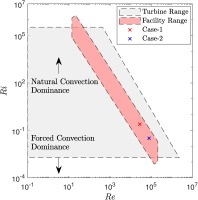### Experiment characteristics.

ParameterCase-1Case-2
GrLg1.86×1081.96×108
ReDh2.75×1047.87×104
Ri(GrLg/ReDh2)0.2460.032

Linear actuators with accuracy of 0.05 mm have been used to traverse the thermocouple probes, which are distributed in axial and circumferential locations. The thermocouple probe houses two K-type thermocouples and a needle to keep the thermocouple beads at a known distance from the heated copper cylinder, as shown in Figure 5. During the measurements, the thermocouple probes were traversed starting from the heated inner cylinder towards the outer cylinder. Step of 0.1 mm was used near the heated wall to be able to capture the near-wall gradients, while relatively larger steps were used away from the walls. Time averaging of unsteady data acquired by National Instruments data acquisition modules was performed over 120 seconds with 50 Hz sampling rate.

Although the heated copper cylinder is covered with a mat black paint, the radiation effect may still be significant. When the heat conduction through the thermocouple sheath tube is ignored, the energy balance applied on a thermocouple bead consists of convective and radiative heat transfer as shown in Equation 2. Measurement or estimation of the effective surrounding temperature, Teff is required to calculate the true fluid temperature, Tf, however it is usually not possible. Therefore, it should somehow be eliminated from the equation. To do so, another thermocouple with different diameter but the same thermophysical properties can be used by relating two energy balance equation for each thermocouple bead (Tagawa and Ohta, 1997; Brohez et al., 2004; Kim et al., 2010).

##### (2)
h(TfTb)=εbσ(Tb4Teff4)

## Numerical methodology

Large Eddy Simulations (LES) have been performed for mixed convection experiments to further investigate the flow field with ANSYS Fluent (ANSYS, 2020). Pressure-velocity coupling is maintained with the SIMPLE algorithm with a segregated manner. Second order scheme has been used for pressure discretisation. A bounded central differencing scheme has been used for momentum and energy. A bounded second order implicit scheme has been chosen for the temporal discretisation. Dynamic Smagorinsky model (Germano et al., 1990) has been employed for sub-grid scale modelling. Large eddy simulations were initialized using the flow field predicted by RANS simulations.

Two conformal structured hexahedral type meshes with 29.6 and 25.3 million nodes have been used for Case-1 and Case-2, respectively. Detailed information on mesh resolutions in wall units is given in Tables 3 and 4.

#### Case-1 mesh resolution.

0<r+<284.1284.1<r+<568.2568.2<r+<852.36
Δx+RiΔθ+RoΔθ+Δrmin+Δrmax+Δrmin+Δrmax+Δrmin+Δrmax+
Case-117.0718.2240.510.293.453.457.581.169.52

#### Case-2 mesh resolution.

0<r+<147.2147.2<r+<1027.61027.6<r+<1366.8
Δx+RiΔθ+RoΔθ+Δrmin+Δrmax+Δrmin+Δrmax+Δrmin+Δrmax+
Case-223.5325.0255.610.314.184.1830.41.2424.3

Time steps of Δt1=2×103 s and Δt2=5×104 s have been used for Case-1 and Case-2, respectively. Both simulations yielded CFL < 0.5. For each case, the simulation had been run until it reached statistically steady state before sampling the unsteady data. Convergence was ensured by monitoring unsteady data at several points in the test section. Initial transients decayed after about 5 flow-through times at each case, then the statistics were collected for 14,000 and 8,000 time steps for Case-1 and Case-2, respectively. The properties of the fluid (air), which was treated as incompressible ideal gas, used for each simulation case are tabulated in Table 5. The fluid properties were evaluated at the ambient condition for each case which read as P1 = 1.003 bar and P1 = 295.65 K for Case-1, and P2 = 1.002 bar and P2 = 294.65 K for Case-2.

#### Fluid properties.

Casek (W/mK)Cp (J/kgK)μ (kg/ms )Prβ (1/K)
Case-10.025871006.9061.84×1050.71443.39×103
Case-20.025791006.8641.83×1050.71463.41×103

Figure 6 shows the amount of resolved turbulent kinetic energy at Section 3 - θ=90 at each case, which indicates that the grid resolution in both cases is sufficient to resolve the significant amount (average of 95%) of the turbulent kinetic energy. Energy spectra of the stream-wise velocity fluctuations are presented in Figure 7 where both cascades agree with the Kolmogorov’s −5/3 slope in the inertial sub-range which further verifies the simulations.

#### Resolved turbulent kinetic energy.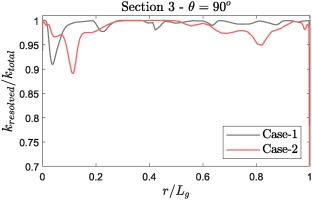#### Energy cascade at r/Lg = 0.9%.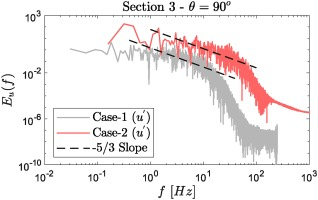### Boundary conditions

The computational domain used for LES is illustrated in Figure 8. As seen in the figure, CFD domain involve a region at upstream of the bellmouth, referred as “entrance region”, to allow boundary layer development over the bellmouth. Mass flow rate for each case has been calculated using the air speed measured at Section 0 by hot-wire anemometry, which read as m˙1 = 0.46 kg/s and m˙2 = 1.31 kg/s for Case-1 and Case-2, respectively. For the inlet boundary conditions, the ambient conditions have been used. Isothermal wall condition has been applied to inner and outer cylinder walls in the test section, and the details of the boundary conditions are given in Table 6. The remaining walls in the domain have been treated as adiabatic walls.

### Computational domain.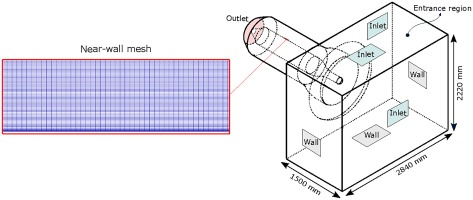### Test section wall boundary conditions.

Test SectionCase-1 (K)Case-2 (K)
Heated inner cylinder422.5426.5
Section 1 Outer CasingLower Half315.5308.1
Upper Half314.5307.1
Section 2 Outer CasingLower Half320.6313.1
Upper Half318.6313.1
Section 3 Outer CasingLower Half316.1311.1
Upper Half317.6311.1

## Heat transfer measurement and uncertainty analysis

This section explains how the heat fluxes from the heated inner cylinder and the Nusselt numbers are calculated from the raw measurement data in this study. In the viscous sub-layer, the heat transfer occurs only through conduction when the radiation is neglected. Temperature field exhibits the same characteristics as the velocity field (Schlichting and Gersten, 2017). Therefore, the temperature gradient remains linear in the viscous sub-layer, and the heat flux from a solid surface to a fluid can be calculated by Fourier’s law (q=kdT/dr). The heat transfer results are presented in Nusselt number form as shown in Equation 3.

##### (3)
Nu=qDi(TiT)k

In the experiments, the temperature gradients were captured using small traverse stepping (0.1 mm) near the heated wall. The near-wall measurement points in the radial direction at each traverse location were fitted to a straight line, and the slope of the each line was used to calculate the heat flux. Fittings were performed using linear regression analysis.

Figure 9 shows the near wall temperature gradient at θ=90 at Section 3 (the other locations are not shown for brevity). The measurement and LES of Case-1 points used in the linear fittings are highlighted. Straight lines created using the best fit are also illustrated, which are used for evaluating the goodness of the fit, R2.

##### Figure 9.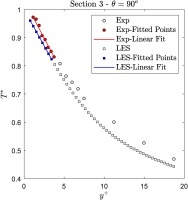In Figure 10, how the experimentally and numerically calculated Nusselt number varies depending on the end point selection is shown. In order to judge the goodness of the fits, R2 values corresponding to both the experiment and LES are also presented in Figure 10 with respect to the right-hand y-axis. The selected LES data points follow a straight line up to y+5 as seen in Figure 9. Figure 10 confirms the goodness of the LES data point fit. Looking at the experimental data points, the R2 values vary remarkably depending on the end point selection since the measurement points do not align as straight as LES data due to the experimental uncertainties. The selected experimental end point is at y+4 which is close to the viscous sub-layer limit at y+=5. LES R2 values also confirms the adequacy of the location of the selected point as R2 starts deviating from R2=1 after y+4.

#### Nusselt number and R2 variation.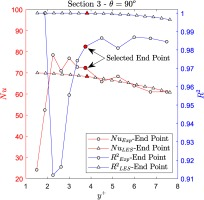The experimental heat flux calculation requires detailed analysis at each measurement point since the viscous sub-layer thickness varies around the heated inner cylinder. In addition, the number of points used for line fitting may change remarkably, which consequently leads to the different uncertainties in Nusselt number around the inner cylinder as shown in Figure 11. The associated bias errors for each variable used in Nusselt number calculation are given in Table 7. The uncertainty analysis has been conducted using root sum square method (Moffat, 1988) as shown in Equation 4. The number of points used for the heat flux calculation effects the uncertainty in Nusselt number calculation remarkably since the the heat flux term, q includes the temperature gradient term where Δr is crucial. The uncertainty due to the Δr term increases greatly when only few points are used for the linear fit since the size of Δr converges to the accuracy of the traverse system. Therefore, a great attention must be paid when calculating the experimental heat flux. To illustrate how significantly Nusselt number uncertainty may vary, the variation in the present study is shown in Figure 11.

#### Uncertainty variation at Section 3.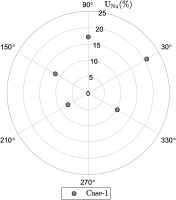#### Bias error of each variable.

VariableUncertaintyVariableUncertainty
Traverse Thermocouple±0.5KHeated Cylinder Diameter±1mm
PID Thermocouple±0.5KTraverse±0.05mm
##### (4)
BNu=n(NuDiVn)2BVn2

Figure 12 indicates the distance from y+=5 around the inner cylinder in both Case-1 and Case-2. Since the large thermal plume does not form in Case-2 due to the strong cross-flow, the viscous sub-layer is much thicker in Case-1 than Case-2. The relatively thin viscous sub-layer in Case-2 has reduced the accuracy of the heat flux calculations due to the insufficient number of data points to be fitted. As previously mentioned, the thermocouple is placed 0.2 mm away from the wall. Considering the size of the thermocouple bead (DS=0.285mm), the first measurement has been ignored to avoid wall proximity effects on the temperature readings, therefore the first measurement point is located 0.3 mm away from the wall. For instance, looking at y+=5 location in Case-2 which reads ∼0.7 mm, the remaining 0.4 mm was not enough to perform multiple thermocouple traversing, and therefore the accurate heat flux calculations could not be performed for Case-2.

#### Sublayer thicknesses at Section 3.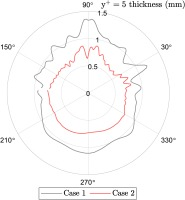## Results and discussion

### Temperature profiles

Temperature profiles are presented in a non-dimensional form as T=((T¯T)/(TiT)). Figure 13 illustrates the qualitative comparison of the flow fields predicted by LES for buoyancy dominant Case-1 and forced convection dominant Case-2. The most prominent difference between the two cases is the growing thermal plume development at the top. In Case-1, the buoyancy forces are strong enough to cause upward flow movement at the top of the inner cylinder. In Case-2, on the other hand, the thermal plume is not formed due to the strong cross flow, therefore leading to more uniform thermal boundary layer thickness around the inner cylinder than Case-1. The lower portion of the annulus in both cases resembles each other with thin laminar boundary layer as also reported in the study of Murat et al. (2021).

### Time-averaged temperature contours.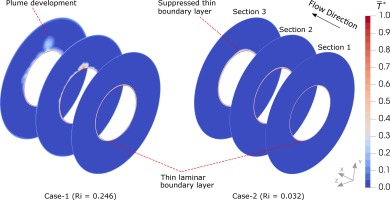Time-averaged non-dimensional temperature profiles predicted by LES are compared against the experimental data in Figure 14 for Case-1 at Section 1, 2 and 3. At Section 1, the thin temperature boundary layer is present around the inner cylinder, indicating that the buoyancy effect is not strongly pronounced at this section. Good agreement between numerical and experimental results has been achieved at Section 1. As the fluid progresses, the development of the boundary layer becomes noticeably distinguishable at the top of the annulus at θ=90 at Section 2. The thickening of the boundary is also present at both sides at θ=30 and 150. The lower portion of the annulus still exhibits a thin boundary layer. Although LES has over-predicted temperature profile at θ=90, there has been satisfactory agreement between LES and the experiment. Towards the downstream of the test section, the thermal plume is further developed at Section 3, resulting in the thickest boundary layer at θ=90. The buoyancy effect is also present at θ=30 and 150 with thicker boundary layers compared to those at Section 1 and 2. The lower portion of Section 3 resembles Section 1 and 2 with a thin boundary layer caused by the buoyancy forces forcing the flow move up against the bottom of the inner cylinder. Overall, LES results agree well with the experimental results at Section 3.

### Time-averaged temperature profiles of Case-1 (Ri = 0.246).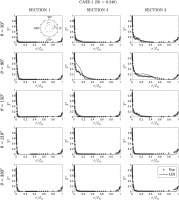Figure 15 shows the temperature profiles from LES and the experiment for Case-2. As opposed to Case-1, Case-2 represents strong momentum of the fluid in the axial direction which reduces the effect of the buoyancy. At Section 1, there is a uniform temperature boundary layer around the inner cylinder which is well predicted by LES. At Section 2, the boundary layer is slightly thickened around the inner cylinder, however the temperature profiles at all measurement locations are very similar, which elucidates the dominance of the forced convection. This flow behaviour can also be seen in Figure 13 where there is no sign of clear plume development. At the upper portion of the annulus at Section 3, the temperature boundary boundary layer is slightly thicker than the lower portion. However, the thermal plume is still not present, therefore the temperature profiles in the annulus are not radically different . Overall, LES has exhibited a good agreement with the experimental data at all sections in Case-2.

### Time-averaged temperature profiles of Case-2 (Ri = 0.032).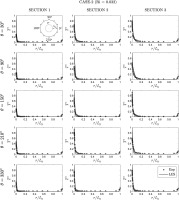### Flow field

The flow field in the annulus can be investigated with the iso-surfaces and their footprints on the heated inner cylinder as illustrated in Figure 16 where the iso-surfaces of the second invariant of velocity (Q=0.5(W2S2)) and the time-averaged wall shear stress on the inner cylinder are shown for each case. In the upstream of the inner cylinder, Case-1 exhibits laminar flow region where the buoyancy is not strongly pronounced in the upper half. As the flow progresses, hairpin-like vortices are formed which causes early transition to turbulence and the the thermal plume lift-off at θ=90. This can also be observed in the reduced wall shear stress at the top of the inner cylinder in 16b. On the sides of the inner cylinder between θ=070 and θ=110180, some of the low momentum fluid near the heated inner cylinder lifts-off due to buoyancy and is stretched towards the downstream by the cross-flow. After the detachment, the fluid shows tendency to move downwards due to a decrease in the temperature, and then re-join the near-wall low-momentum fluid that moves upwards. Therefore, longitudinal helical structures, which enlarge along the stream-wise direction, form as shown in Figure 16a. These flow structures can be tracked by looking at their footprints on the inner cylinder as illustrated in Figure 16b where the stretched streaks are seen.

### (a) Iso-surfaces of second invariant of velocity, (b) Time-averaged wall shear stress around the inner cylinder.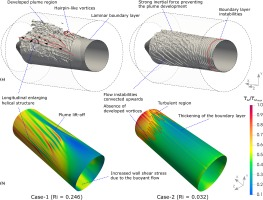As opposed to Case-1 which represents a strong buoyancy influence in the flow field, Case-2 exhibits strong cross-flow influence which minimises the buoyancy effect. This phenomenon can be observed by looking at Case-2 iso-surfaces in 16a where the thermal plume is not formed at θ=90 due to the strong inertial force. Boundary layer instabilities are present in the upstream of the inner cylinder due to the relatively high speed flow. Towards the downstream, the low momentum buoyant flows near the inner cylinder are convected to the top of the inner cylinder. However, these near-wall fluid movements remain as instabilities as seen in Figure 16 where there is no sign of developed vortices. In the downstream, the turbulent region exists as seen in locally increased/decreased wall shear stress.

Although the lower portion of the annulus remains laminar with both cases, there is a locally increased wall shear stress at the bottom of the inner cylinder in Case-1 due to the buoyant forces pushing the flow upwards against the inner cylinder which suppress the growth of the boundary layer. The shear stress distribution in Case-2 remains relatively uniform until the beginning of the turbulent region.

### Heat transfer

Table 8 shows the detailed information on Nusselt number distribution at circumferential and axial measurement locations, which could be used for validation purposes. For Case-1, experimentally and numerically calculated Nusselt numbers as well as the LES errors are tabulated. Since the experimental heat transfer could not be obtained for Case-2 as described earlier, LES results are presented only for Case-2. Figure 17 illustrates the heat transfer distribution around the circumference at Sections 1–3.

### Inner cylinder Nusselt number comparison.

Section 1Section 2Section 3
θExp-1LES-1E-1 (%)LES-2Exp-1LES-1E-1 (%)LES-2Exp-1LES-1E-1 (%)LES-2
3053.2954.011.3398.5169.9766.81−4.7465.2786.5193.887.8597.35
9040.5341.542.4390.2542.0134.95−20.1946.4772.3470.19−3.0789.73
15052.8553.751.6797.2790.9996.806.0162.93104.11106.011.7899.38
21073.8884.0612.11102.8765.1767.353.2474.7164.1464.460.4978.41
33067.8286.9021.9599.7968.7570.021.8168.8363.3264.591.9767.58

### Heat transfer distribution around the inner cylinder.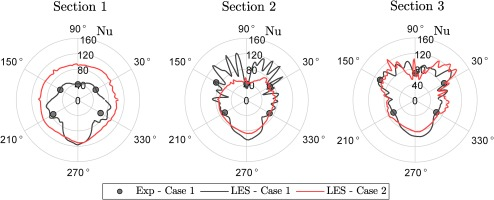There is a heat transfer enhancement at the bottom of the inner cylinder compared to the top with Case-1, indicating that the buoyant flow causes a large temperature gradient (thin boundary layer) whereas Case-2 exhibits a uniform heat transfer around the inner cylinder at Section 1. The heat transfer drop phenomenon between the bottom and top in Case-1 is one of the well-known characteristics of the pure natural convection from a horizontal cylinder (Kenjereš and Hanjalić, 1995), which indicates the dominance of the natural convection. There are greater heat transfer values at each sides of the cylinder than that of the top. This could be attributed to the start of formation of the side helical structures. LES results show good agreement with Case-1 experiment with a maximum error of 21.95% at θ=330.

At Section 2, the Nusselt number alternates remarkably at the upper half of the cylinder in Case-1 due to developed side structures, footprints of which are shown in Figure 16. Conversely, the bottom exhibits more uniform heat transfer rates which are slightly lower than Section 1 since the boundary layer is thicker. It is also interesting note that the minimum heat transfer has occurred at the top, indicating that the thermal plume lift-off has formed a large boundary layer. Although the highest LES error (-20.19%) has occurred at θ=90, the overall trend has been captured at Section 2. The heat transfer distribution around the inner cylinder is still uniform with Case-2 at lower rates than Section 1. This is due to the slightly thicker boundary layer.

Although there are still heat transfer minima/maxima at the upper half of the cylinder at Section 3 in Case-1, the fluctuations are not as strong as those at Section 2 since the flow is more developed at Section 3. Developing helical structures shown in Figure 16a have enhanced the heat transfer rates at both sides of the inner cylinder around 45 and 135. The heat transfer is further reduced at the bottom as the boundary layer progressively thickens which is also valid for Case-2. Comparable heat transfer behaviour at the bottom in Case-1 and Case-2 implies that the lower half between 210 and 330 is not significantly affected by the rate at which the mixed convection occurs. On the other hand, the upper half in Case-2 now demonstrates alternating Nusselt numbers since the flow is in the region where the turbulence occurs as shown in Figure 16b. As seen in Table 8, there is a good agreement between LES and experimental results for Case-1 at Section 3.

## Conclusions

This paper has presented unique experimental data for mixed (natural and forced) type of flows which occur in turbine casing cavities during shut-down regimes. Detailed measurements have been performed in axial and circumferential directions to acquire detailed information in the large majority of the test section. Two experimental campaigns with different buoyancy force dominance have been performed to investigate the mixed convection cases at different rates since the real turbine casings are exposed to various mixed type of flows during shut-down regimes. Heat flux calculations from the thermocouple measurements have been discussed and the limitations due to the viscous sub-layer thickness have been highlighted. These experimental results can be used for the validation purposes and the development of the numerical tools for complex flow interactions in mixed type of flows. Additionally, large eddy simulations have been performed and validated against the experimental data. The scale resolving simulations have provided a great insight into the flow field with distinct flow structures for each case. In Case-1 where the buoyancy was more pronounced, a developed thermal plume region was observed towards the downstream with stretched helical structures at sides. The helical structures were found to cause large alternations in the heat transfer distribution around the inner cylinder. Case-2 where the dominance of the inertial forces was present exhibited almost circumferentially uniform boundary layer thickness. However, the varying enhanced heat transfer on the upper half of the heated inner cylinder was observed in the downstream due to the turbulent region.

These experimental and numerical results should contribute to the fundamental understanding of mixed type of flows in turbine casing cavities, which are of great importance for enabling flexible operations of the future power plants.

## Nomenclature

Symbols

B

Bias error

Cp

Specific heat capacity (J/kgK)

Di/o

Inner/Outer diameter (m)

Dh

Hydraulic diameter (DoDi) (m)

E

Error %

f

Frequency (Hz)

k

Thermal conductivity (W/mK)

g

Gravitational acceleration (m/s2)

h

Heat transfer coefficient (W/m2K)

Gr

Grashof number

Lg

Gap width (m)

Nu

Nusselt number

Pr

Prandtl number

r

R2

Goodness of the fit in linear regression

Re

Reynolds number

Ri

Richardson number

S

Strain rate (1/s)

T¯

Time-averaged temperature (K)

Tb

Teff

Effective surrounding temperature (K)

Tf

True fluid temperature (K)

τ¯ω

Time-averaged wall shear stress (pascal)

U

Uncertainty %

V

Variable

Q

Second invariant of velocity (1/s2)

W

Vorticity magnitude (1/s)

q

Heat flux (W/m2)

u

Velocity (m/s)

uτ

Friction velocity (m/s)

εb

Emissivity

β

Thermal expansion coefficient (1/K)

ν

Kinematic viscosity (m2/s)

θ

Angle () or circumferential direction

σ

Stephan-Boltzmann constant (5.6703×108(W/m2K4))

Acronyms

LES

Large eddy simulation

SGS

Sub-grid scale

HP

High pressure

RANS

Reynolds-averaged Navier-Stokes